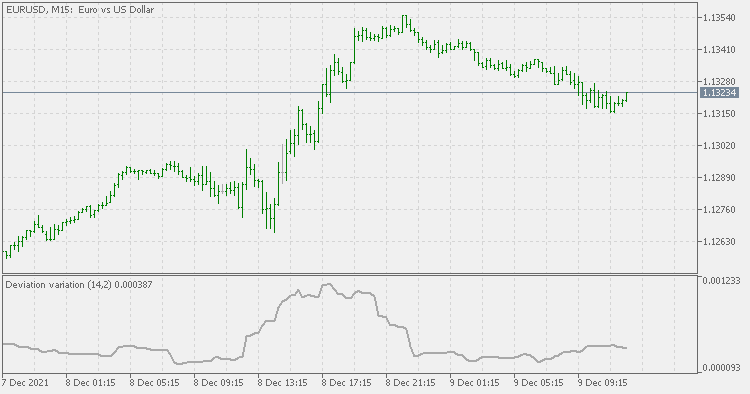Interesting script?
So post a link to it -
let others appraise it
You liked the script? Try it in the MetaTrader 5 terminalMomentum deviation - indicator for MetaTrader 5

Views:
1337
Rating:
Published:
2021.12.09 11:28

This is a variation on the standard deviation

• it is using momentum of price  (instead price itself) for calculation
• it is calculated in a way that it takes minimal CPU load

As far as values are concerned, it is similar to standard deviation. Same as standard deviation it can calculate deviation of anythingComparison : upper is this one, lower is standard deviation

Recommendations:
• You can use it as any standard deviation indicatorBobokus Fibonacci

Select Timeframe to draw Bobokus Fibonacci and add any custom fibonacci levels you wantConsolidation Detector

Draws an equidistant channel to detect rectangular consolidation pattern on chartMomentum deviation bands

Momentum deviation bandsZigZag Color for Line Chart

ZigZag Color Indicator for Line Charts which works on Close prices rather than High low This indicator has only one input parameter which is ExtDepth and its optimized for performance